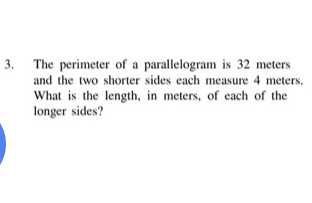### ¿Todavía tienes preguntas de matemáticas?

Pregunte a nuestros tutores expertos
Algebra
Pregunta3. The perimeter of a parallelogram is $$32$$ meters and the two shorter sides each measure $$4$$ meters. What is the length, in meters, of each of the longer sides?